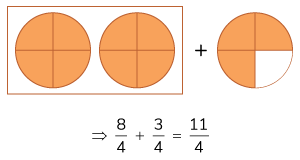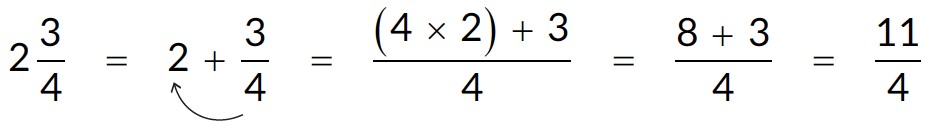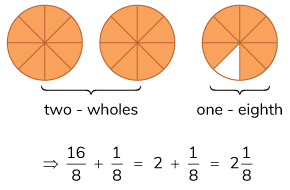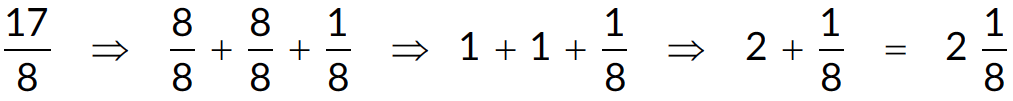# Improper and Mixed Fractions

Go back to  'Fractions'

## Types of Fractions

Proper fraction: A fraction whose numerator is less than its denominator. For example, \begin{align} \frac {3}{12},\;\frac {2}{3} \end{align} are proper fractions. Numerically, a proper fraction is always less than $$1.$$

Improper fraction: A fraction whose numerator is greater than its denominator. For example, \begin{align} \frac {5}{2},\;\frac {8}{7},\;\frac {4}{9} \end{align} are improper fractions. Numerically, an improper fraction is always greater than $$1.$$

Mixed fraction: A fraction that is written as a combination of a natural number and a proper fraction. For example, \begin{align} 1\frac {2}{3},\;3\frac {1}{3} \end{align} are mixed fractions. Numerically, a mixed fraction is always greater than $$1.$$ Also, any mixed fraction is an improper fraction.

Unit fraction: A unit fraction is a fraction in which the numerator is equal to $$1.$$ For example, \begin{align} \frac {1}{3},\;\frac {1}{4} \end{align} are unit fractions.

## Conversion of fractions

A mixed fraction can be converted into an improper fraction and vice versa.

A mixed fraction can be converted into an improper fraction in one of the following ways,

a)  Let us consider the mixed fraction \begin{align} 2\frac {3}{4}. \end{align} This can be written as \begin{align} 2+\frac {3}{4}. \end{align} Now representing this visually, we getb)  This can also be done using the cross multiplication method (also called as the LCM method.)An improper fraction can be converted into a mixed fraction in one of the following ways,

a)  Let’s consider the fraction \begin{align} \frac {17}{8}. \end{align} It can be represented visually as given,b)  This can also be done as follows. (Decomposing $$17$$ as $$8 + 8 + 1$$)## Unlike fractions

Unlike fractions are those fractions whose denominators are different. For example, \begin{align} \frac {2}{3} \end{align} and \begin{align} \frac {3}{4} \end{align} are unlike fractions.

### Comparing unlike fractions

To compare unlike fractions, we use the idea of the Least Common Multiple (or the LCM)! In other words, we calculate the LCM of the denominators of the two fractions.

Example: Compare \begin{align} \frac {2}{3} \end{align} and \begin{align} \frac {3}{4} \end{align}

In the case of the fractions \begin{align} \frac {2}{3} \end{align} and \begin{align} \frac {3}{4}, \end{align}  we take the LCM of $$3$$ and $$4$$ which is $$12.$$

So, the fractions equivalent to the original fractions are \begin{align} \frac {2}{3} \end{align} and \begin{align} \frac {3}{4}. \end{align}

\begin{align} \frac {2}{3} = \frac {2 \times 4}{3 \times 4} = \frac {8}{12} \end{align} and \begin{align} \frac {3}{4} = \frac {3 \times 3}{4 \times 3} = \frac {9}{12} \end{align}

\begin{align} \frac {8}{12} \lt \frac {9}{12} \end{align}

\begin{align} \frac {2}{3} \lt \frac {3}{4} \end{align}

## Tips and Tricks

• Tip: Using language that students can relate to makes the understanding of mixed and improper fractions easier. For example, describe \begin{align} \frac {7}{4} \end{align} as having $$7$$ pieces from a cake shop that cuts their cakes only in $$4$$ pieces each. This way students will make the connection that to have $$7$$ pieces, that must have a full cake and $$3$$ more pieces.

• Tip: Give a few examples of improper and mixed fractions and ask students to plot them on a number line. Even doing this for $$5$$-$$6$$ examples conveys that idea that mixed and improper fractions are numbers that are greater than $$1$$ and can be plotted on the number line.

### Common mistakes or misconceptions

• Children often don’t see a mixed fraction and it’s improper form as equal numbers. Because mixed fractions have a whole number part, it is assumed to be larger.
• Children often can’t plot improper fractions and mixed fractions on the number line.
This happens because they have seen fractions only as shapes. They may understand fractions as a part of the whole but don’t see them as numbers that can be plotted on the number line. Given that improper fractions are larger than 1, there is an additional challenge in plotting these numbers.

## Solve for improper fractions

Q1. Convert mixed fraction into an improper fraction.

a.  \begin{align} 3\frac {5}{6} \end{align}

b.  \begin{align} 3\frac {2}{3} \end{align}

c.  \begin{align} 6\frac {2}{7} \end{align}

More Important Topics
Numbers
Algebra
Geometry
Measurement
Money
Data
Trigonometry
Calculus
More Important Topics
Numbers
Algebra
Geometry
Measurement
Money
Data
Trigonometry
Calculus
Learn from the best math teachers and top your exams

• Live one on one classroom and doubt clearing
• Practice worksheets in and after class for conceptual clarity
• Personalized curriculum to keep up with school

0